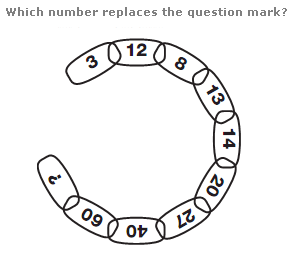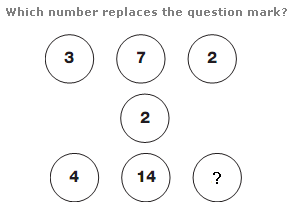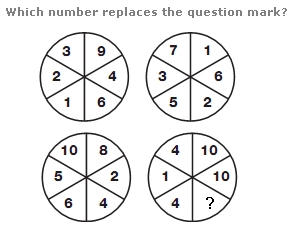# Puzzles - Number puzzles

### Exercise :: Number puzzlesAnswer : 93 Explanation : Starting with the top segment, and moving clockwise, add the numbers in the first two segments together and subtract 7 to give the next number around.Answer : 6 Explanation : Starting with the numbers in the top row, left to right, multiply each one by 2 and write the results in the lower row, from right to left.Answer : 8 Explanation : Starting with the numbers in the top two circles, add numbers in the left hand segments of each circle, and put these values in the left hand segments of the lower left circle. Put the difference between segments in the right hand halves of the two top circles in the right hand half of the lower left circle. Repeat this pattern for the lower right circle, but putting the difference between numbers in the left hand half of the top two circles in the left hand half of the lower right circle, and the sum of the right hand half of the top two circles in the right hand half of the lower right circle.